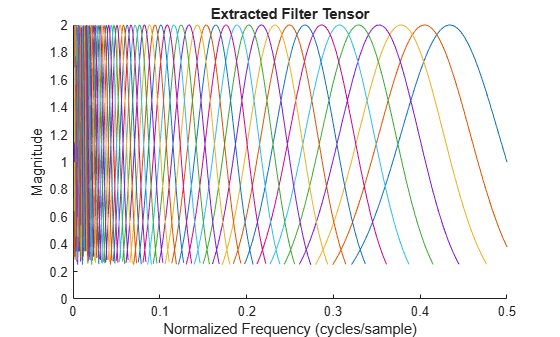# cwtfilters2array

Convert CWT filter bank to reduced-weight tensor for deep learning

Since R2022b

## Syntax

``[psifvec,filteridx] = cwtfilters2array(wfb)``
``[psifvec,filteridx] = cwtfilters2array(___,thresh)``
``[psifvec,filteridx] = cwtfilters2array(___,IncludeLowpass=tf)``

## Description

````[psifvec,filteridx] = cwtfilters2array(wfb)` converts the CWT filter bank `wfb` to a reduced-weight CWT filter tensor `psifvec` for deep learning. `filteridx` is a bookkeeping matrix.```

example

````[psifvec,filteridx] = cwtfilters2array(___,thresh)` uses `thresh` to extract the significant values from each of the CWT filters in `wfb`. By thresholding, you can significantly reduce the number of learnable parameters in the filter bank.```
````[psifvec,filteridx] = cwtfilters2array(___,IncludeLowpass=tf)` specifies whether to include the lowpass (scaling) filter in `psifvec`.```

## Examples

collapse all

Create a CWT filter bank appropriate for deep learning. Specify a signal length of 2048 samples. Use the default Morse wavelet.

```len = 2048; fb = cwtfilterbank(SignalLength=len,Boundary="periodic");```

Extract the reduced-weight CWT filter tensor from the filter bank. Specify a threshold of `1/4`.

`[psifvec,filteridx] = cwtfilters2array(fb,1/4);`

Use the bookkeeping matrix `filteridx` to plot the filters in `psifvec`.

```fBins = (0:len-1)/len; hold on for k=2:size(filteridx,1) indX = filteridx(k,1:2); indY = filteridx(k,3:4); rangeX = indX(1):indX(2); rangeY = indY(1):indY(2); plot(fBins(rangeX),squeeze(psifvec(rangeY))) end title("Extracted Filter Tensor") xlabel("Normalized Frequency (cycles/sample)") ylabel("Magnitude") hold off ylim([0 2])```## Input Arguments

collapse all

CWT filter bank, specified as a `cwtfilterbank` object. `wfb` must have `Boundary="periodic"`. For more information, see `cwtfilterbank`.

Threshold to apply to the CWT filters in `wfb`, specified as a real scalar. `cwtfilters2array` uses `thresh` to extract the significant values from each of the CWT filters in `wfb`. The filters are normalized so that the peak value for each filter is 2

• Smaller values of `thresh` result in more values being retained from the CWT filters and therefore less weight reduction.

• Larger values of `thresh` result in more weight reduction and more divergence between the deep learning CWT and transforms computed with the full filter bank.

• Any threshold less than `realmin`, the smallest positive normalized floating-point number in double precision, is set to `realmin` for extracting the filter values.

Setting `thresh` to a value which results in no values being retained for any individual filter results in an error.

Data Types: `single` | `double`

Include lowpass filter, specified as a numeric or logical `1` (`true`) or `0` (`false`). Specify `true` to include the lowpass (scaling) filter in `psifvec`. The size of `filteridx` increases to Nfilt+2-by-4, where Nfilt is the number of filters in the CWT filter bank `wfb`. The meta information for the scaling filter is included in the final row of `filteridx`.

Data Types: `logical`

## Output Arguments

collapse all

Reduced-weight CWT filter tensor, returned as a 1-by-1-by-Nr tensor, where Nr is the number of filter values greater than `thresh`.

Data Types: `double`

Bookkeeping matrix that describes `psifvec`, returned as an Nfilt+1-by-4 matrix, where Nfilt is the number of filters in the CWT filter bank `wfb`.

• The first row of `filteridx` is ```[1 Nf 0 0]```, where Nf is the number of frequency bins, or equivalently the number of time points in the wavelet filter. Nf is equal to the `SignalLength` property of `wfb`.

• For rows 2 through Nfilt+1, the first two columns of `filteridx` contain the beginning and ending frequency bins for the corresponding wavelet filters ordered by decreasing center frequency. The third and fourth columns of `filteridx` contain the beginning and ending indices of the corresponding filter in the reshaped tensor, `psifvec`.

Use `array2cwtfilters` to reconstruct an approximation to the wavelet filter bank from `psifvec` and `filteridx`.

Data Types: `uint32`

## Version History

Introduced in R2022b